# Introduction to Algebraic Geometry

(Math 665, fall 2001)
Donu Arapura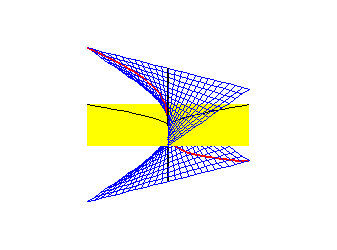Blow up

(If this doesn't look right, use the html version.)

## Learning the language

In order to get a feeling for what algebraic geometry is, let's to go through some examples. Consider the space of 2x2 matrices over a field k with trace 0. This consists of matrices of the form $\left(\begin{array}{cc}\hfill x\hfill & \hfill y\hfill \\ \hfill z\hfill & \hfill -x\hfill \end{array}\right)$ which can be identified with points (x,y,z) of three dimensional affine space ${A}^{3}$ . In these coordinates the determinant $\mathrm{det}\left(x,y,z\right)=-{x}^{2}-\mathrm{yz}$ . The determinant is an algebraic map, and the fibers

${X}_{t}={\mathrm{det}}^{-1}\left(t\right)=\left\{\left(x,y,z\right)|\mathrm{det}\left(x,y,z\right)=t\right\}\text{}$

are algebraic subsets of ${A}^{3}$ . These are in fact varieties since the polynomials ${x}^{2}-\mathrm{yz}-t\text{}$ are irreducible for each t in k.

Let's study the geometry of these sets. Suppose k=C, then I claim that ${X}_{t}$ is isomorphic to ${X}_{1}$ whenever t is nonzero. To see this, choose a such that ${a}^{2}=t$ , then the map $\left(x,y,z\right)\to \left(\mathrm{ax},\mathrm{ay},\mathrm{az}\right)$ defines an isomorphism between $X{}_{1}$ and ${X}_{t}$ ; this can be checked by comparing coordinate rings. ( This works even if C is replaced by an algebraically closed field. But it fails in general. For example when k =R, ${X}_{t}$ is connected in it's usual topology while ${X}_{-1}$ isn't.) I claim that ${X}_{1}$ is a homogeneous space which implies that any point looks like any other point. To see this, observe that the algebraic group ${\mathrm{SL}}_{2}\left(C\right)$ acts on ${X}_{1}$ by matrix conjugation, and that this is a transitive action.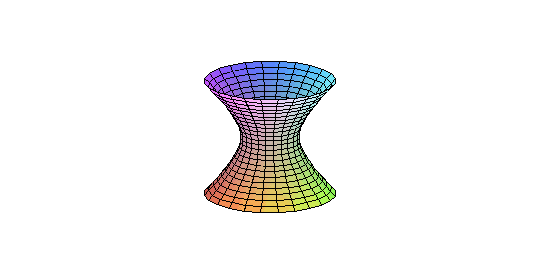${X}_{1}$

${X}_{0}$ is the space 2x2 matrices with zero trace and zero determinant. It follows by the Cayley-Hamilton theorem that this is precisely the set of nilpotent matrices. The zero matrix is a singular point of this set, while the other points are nonsingular. It follows that ${X}_{0}$ is not homogenous, and therefore ${X}_{0}$ is not isomorphic to ${X}_{1}$ . Alternatively, these cannot be isomorphic since all the points of ${X}_{1}$ are nonsingular.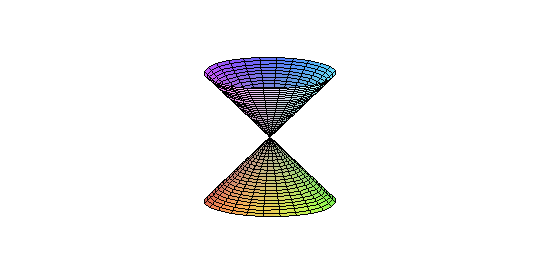${X}_{0}$

What we've been doing so far is affine geometry. We get a little more insight into the structure of these sets by doing projective geometry. A matrix in ${X}_{-1}$ has 1 and -1 as its eigenvalues. The eigenvectors span two distinct lines in ${C}^{2}$ . Conversely, a pair of distinct lines determines an element of ${X}_{-1}$ . Thus we have a bijection, and in fact isomorphism, between the ${X}_{-1}$ and the product of two copies of the projective line minus the diagonal. Thus ${X}_{-1}$ is a so called doubly ruled surface. These rulings, which are fibers of the projections onto the factors, are embedded as lines in ${A}^{3}$ . In fact, after a linear change of coordinates the embedding of ${X}_{-1}$ into ${A}^{3}$ extends to the Segre embedding of ${P}^{1}×{P}^{1}$ to ${P}^{3}$ .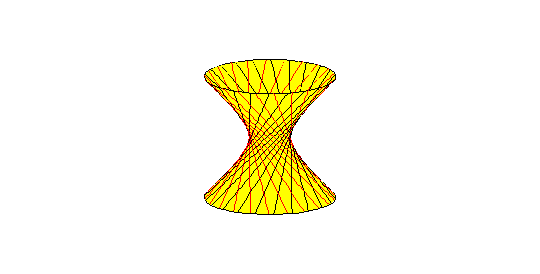Double ruling (red and black lines)

It follows from above that ${X}_{-1}$ is birational to the ${P}^{1}×{P}^{1}$ and therefore to ${A}^{2}$ . However, it is not isomorphic to ${A}^{2}$ . This is because the coordinate ring $A{\left(A}^{2}\right)=k{\left[x}_{1},{x}_{2}\right]$ is a unique factorization domain, while $A{\left(X}_{-1}\right)=k\left[x,y,z\right]/\left(1-{x}^{2}-\mathrm{yz}\right)$ isn't (the image of yz can be factored in two different ways).

****

The second example, which is the one indicated in the picture at the top, is the blow up of the affine plane. This consistis of a quasiprojective variety Bl and a morphism $p:Bl\to {A}^{2}$ , where Bl consists of pairs (x,L) where x is point in ${A}^{2}$ and L point in ${P}^{1}$ containing x, and p(x,L) = x. The morphism p induces isomorphism ${p}^{-1}{\left(A}^{2}-\left\{\left(0,0\right)\right\}\right)\to {A}^{2}-\left\{\left(0,0\right)\right\}$ . Therefore p is a birational equivalence. However, p is not an isomorphism, since the preimage $E={p}^{-1}\left(0,0\right)$ is ${P}^{1}$ rather than a point. Bl can be described as a union of two affine varieties, ${\mathrm{Bl}}_{1}=\left\{\left(x,y,t\right)|y=\mathrm{xt}\right\}$ and ${\mathrm{Bl}}_{2}=\left\{\left(x,y,u\right)|x=\mathrm{yu}\right\}$ glued via $\left(x,y,t\right)\to \left(x,y,1/t\right)$ . ${\mathrm{Bl}}_{1}$ is blue surface depicted above.

The curve $C=\left\{\left(x,y\right)|{y}^{2}={x}^{3}\right\}$ in ${A}^{2}$ is singular at (0,0). Its preimage under p, called its total transform, is the union of E with a curve ${C}_{2}$ (the red curve). ${C}_{2}$ , which can be described as the closure of the preimage of C-{(0,0)}, is called the strict transform. This curve lies in ${\mathrm{Bl}}_{1}$ and is given parametrically by $x={s}^{2},$ $y={s}^{3}$ , $t=s$ . The map ${A}^{1}\to {C}_{2}$ given by $s\to \left(x,y,t\right)$ is an isomorphism, since $t\to s$ is the inverse, therefore ${C}_{2}$ is smooth. Since ${C}_{2}$ and C are isomorphic away from (0,0), they are birational. The map on coordinate rings $A\left(C\right)=k\left[x,y\right]/\left({y}^{2}-{x}^{3}\right)\to A{\left(A}^{1}\right)=k\left[s\right]$ is given by $x\to {s}^{2}$ , $y\to {s}^{3}$ , so A(C) can be identified with the subring of k[s] generated by ${s}^{2}$ and ${s}^{3}$ . ${C}_{2}\to C$ is an example of resolution of singularities.

## Books

Harris is the text for this class (although I won't follow it too closely).

1. M. Atiyah,I. MacDonald, Commutative Algebra
2. D. Cox, J. Little, D. O'Shea, Ideals, Varieties and Algorithms
3. D. Cox, J. Little, D. O'Shea, , Using Algebraic Geometry
4. D. Eisenbud , Commutative Algebra
5. J. Harris, Algebraic geometry: a first course
6. R. Hartshorne, Algebraic geometry*
7. D. Mumford, Red book of varieties and schemes*
8. I. Shafarevich, Basic Algebraic Geometry
The starred references would be a little heavy going as an introduction.

## Software

If you're comfortable with computers, there are a couple of software packages that might help with working out examples. For general purpose algebraic manipulation, graphics etc. there's Maple or Mathematica. (I tend to use Maple since it's is available on most of our machines including all our Suns.) Some examples of the use of Maple for algebro-geometric calculations can be found in the books of Cox et. al. Here, I'll just give the code for generating the graph of the blow up at the top of this page.
with(plots): Bl := plot3d([x,x*t, t], x=-1..1, t=-1..1, style=WIREFRAME, color =blue):

A2 := plot3d([x,y,0], x=-1..1, y=-1..1, color=yellow, style=PATCHNOGRID):

E := spacecurve([0,0,t], t=-1..1, color=black, thickness=2):

C := spacecurve([s^2, s^3, 0], s=-1..1, color=black, thickness=1):

C2 := spacecurve([s^2,s^3,s], s=-1..1, color=red, thickness=2): display({Bl,A2, E, C, C2});

****

For doing calculations in algebraic geometry and commutative algebra, Grayson and Stillman's Macaulay2 program is more powerful than Maple or Mathematica. Documentation is available on the web or as a (very big) pdf file. To get a sense for what it can do, let's consider some simple examples. Start the program by typing M2 in a terminal window of one of our Suns or whatever machine you're using. (If you plan to do anything serious, you'll need to learn how to run it under emacs.)

If this doesn't work then read this.

1. As a first example, let's check a special case of the Cayley-Hamilton theorem. At the prompt (i1:) type

R = QQ[x_1..x_4]
This sets up a polynomial ring over Q (= QQ in Macaulay) in 4 variables. Next define the ideal I generated by det and trace of the "universal" 4x4 matrix M by typing
M = matrix{{x_1,x_2},{x_3,x_4}}

D = det M

T = trace M

I = ideal {D, T}

The algebraic set V(I) in ${A}^{4}$ (= space of 2x2 matrices) is the set of matrices with det= trace = 0 Let Nilp be the set of matrices whose square vanishes. The entries of ${M}^{2}$ generate an ideal J which can be constructed by
J = ideal M^2
Then Nilp = V(J). By the Cayley-Hamilton theorem, V(I) coincides with Nilp as well. Let's check this directly. In order verify V(I) = V(J) (over any algebraically closed field of char. 0), it's enough, by the Nullstellensatz, to check the equality of the radicals of I and J:
Exercise: check whether I = J? If not, then what goes wrong?

****

2. Next, let's check that the hypersurface $f={z}^{2}-\left(y-1\right){\left(y}^{2}-x\right)=0$ in ${A}^{3}$ has exactly one singular point at (1,1,0) over an algebraically closed field of large positive characteristic (Macaulay2 computes more efficiently over finite fields).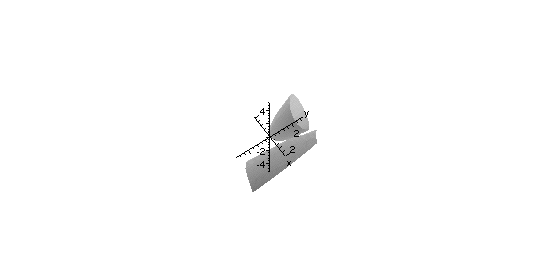We need to clear the previous use of x before defining the new objects
erase symbol x

R = ZZ/31991[x,y,z]

f = z^2 -(y-1)*(y^2-x)

The graph (which is a bit misleading) suggests that the hypersurface might be reducible. We can check that it's irreducible over the prime field Z/31991 by trying to factor it:
factor f
Next define the ideal Jac generated by f and its partials.
Jac = ideal { f, diff(x,f), diff(y,f), diff(z,f)}
The singular locus of f is precisely V(Jac). It is enough by the Nullstellensatz to check the radical of Jac is the maximal ideal (x-1,y-1,z)
****

3. For the last example, let's identify ${A}^{8}$ with space of 2x4 matrices. Consider the morphism $P:{A}^{8}\to {A}^{6}$ given by sending a matrix to the vector of its 2x2 minors (in some order). Let X be the image of F, and let's find I(X). (In more geometric terms, we're determining the Pluecker equations for the Grassmanian of 2-planes in affine 4-space.) Let R and S be the coordinate rings of these affine spaces.

erase symbol x

erase symbol y

R = QQ[x_1..x_8]

S = QQ[y_1..y_6]

Let p be homomorphism from S -> R determined by P.
M = genericMatrix(R,x_1,2,4)

M2 = exteriorPower(2,M)

p = map(R,S, M2)

Then I(X) = ker(p)
ker p
When we're finished, type
quit
Back to beginning.

--------------------------------------------------------

This information is relevant for people usings Suns at Purdue Math only:

If "M2" doesn't work, it means that the command is not on your path. You'll need to add it. Unfortunately, there are two different locations in our dept. depending on the version of the operation system.

Type
uname -r
If it's 5.5.1, use /opt/Macaulay2-0.8.60/bin If it's 5.8, use /pkgs/macaulay2-8.60/bin/ If all else fails, you can use my personal version /.amd_mnt/banach//export/u7/dvb/Macaulay2/bin on a Solaris 5.8 machine.

Mathematics may be timeless, but this information certainly isn't. If things don't work in the future, check here again. (Note: I'm currently, as of fall '02, running linux on my office machine, so I probably won't bother updating the Sun stuff.)

--------------------------------------------------------

This web page was created on a Mac running yellowdog linux and MacOS9, with amaya, emacs, itex2html, mozilla, Macaulay2, Maple, GifBuilder (the latter two running under MacOS). The equipment was purchased through an NSF grant.

Last revision 11/08/02